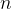# Thread: For 0\le n\le 10, find a formula for p_n, the payment in year n on a loan of 100000 d

1. ## For 0\le n\le 10, find a formula for p_n, the payment in year n on a loan of 100000 d

For, find a formula for, the payment in yearon a loan of 100000 dollars. Interest is 5 percent per year, compounded annually, and payments are made at the end of each year for 10 years. Each payment is 10000 dollars plus the interest on the amount of money outstanding.

i got 10000 + (100000-10000n)(.05)^(n+1), but it's wrong

2.Originally Posted by ewkimchiFor, find a formula for, the payment in yearon a loan of 100000 dollars. Interest is 5 percent per year, compounded annually, and payments are made at the end of each year for 10 years. Each payment is 10000 dollars plus the interest on the amount of money outstanding.

i got 10000 + (100000-10000n)(.05)^(n+1), but it's wrong
The formula for loan amortization is given at Amortization Calculation Formula and Payment Calculator

It is $\displaystyle A= P\frac{r(1+r)^n}{(1+r)^n-1}$

Here, P is the original amount of the loan, n is the number of payments, and r is the interest rate per payment period. For this problem, P= 100000, n= 10, and r= .05.

If you were making monthly payments, n would be 120 and r would be .05/12.

### For 1≤n≤101n10, find a formula for pnpn, the payment in year nn on a loan of 110000 dollars. Interest is 8 percent per year, compounded annually, and payments are made at the end of each year for 10 years. Each payment is 11000 dollars plus the intere

Click on a term to search for related topics.

#### Search Tags

0le, formula, loan, nle, payment, year